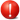# xlf | GetArrDim VBA function

## 1. GetArrDim - about the function

Description: returns an integer value of the number of dimensions in a VBA array. Not an array returns the value -99

Syntax: GetArrDim(inArr)

Arguments: compulsory - inArr, a valid VBA array, as shown by the array brackets "()" in figures 1 and 2 (Type column).Array of Variants - the GetArrDim procedure is only valid in the case a single array, identified by one set of brackets such as inArr(). It will not function with multiple arrays, such as arrays of optional variants, returned by the Paramarray array keyword such as inASrr()()()

## 2. VBA code

### 2.1 GetArrDim

Code 1: Function GetArrDim - returns the number of dimensions in a VBA array
Option Explicit
Option Base 1

' ================================
Function GetArrDim(inArr As Variant) As Integer
Dim i As Integer

GetArrDim = -99
If Not IsArray(inArr) Then Exit Function

On Error Resume Next
Err.Clear
Do While IsNumeric(UBound(inArr, i + 1))
If Err.Number = 9 Then Exit Do
i = i + 1
Loop
GetArrDim = i
On Error GoTo 0

End Function


1. Line 5: Function declaration statement - if inArr is an array, then return the index number of the highest dimension. For example a 2D array returns 2, a 3D array returns 3, a 4D array returns 4, ...
2. Line 9: If inArr is not an array, then exit function and return value -99 assigned in line 8
3. Line 11: Suppress error VBA message - "Run-time error '9': Subscript out of range" that occurs when the UBound dimension subscript does not exist. Resume execution at the next code statement, line 12
4. Line 12: Clear any existing errors from Err object. The Err object has properties with values representing the most recent error
5. Line 13 to 16: While the upper index number of the dimension is a number (IsNumeric returns a Boolean) keep incrementing the dimension numbers at line 15

### 2.2 Test the GetArrDim function

Code 2: Macro TestGetArrDim
' ================================
Sub TestGetArrDim()

' Declare arrays
Dim Arr() As Variant    ' an array of variants
Dim One(3) As Double
Dim Two(3, 4) As String
Dim Three(3, 4, 5) As Integer

Dim A As Variant        ' a variant array
Dim At As Variant

A = Array(1, 2, 3, 4)
At = WorksheetFunction.Transpose(A)

' Group one === figure 1
Debug.Print Time & " ===" & vbNewLine
Debug.Print "Arr: " & GetArrDim(Arr)        ' Arr: 0
Debug.Print "One: " & GetArrDim(One)        ' One: 1
Debug.Print "Two: " & GetArrDim(Two)        ' Two: 2
Debug.Print "Three: " & GetArrDim(Three)    ' Three: 3

' Group two === figure 2
Debug.Print "A: " & GetArrDim(A)            ' A: 1
Debug.Print "A transpose: " & GetArrDim(At) ' A transpose: 2

Stop    ' Halt execution - to view Locals window

End Sub


VBA terminology Description
Err.Number
(Err object, Number property)
Returns or sets a value specifying an error number
Error numbers
9 Subscript out of range
IsNumeric
(Function)
Returns a Boolean TRUE if an expression can be evaluated as a number
On Error{Resume Next}
(Statement)
Unstructured error handling. The Resume Next part specifies that control goes to the statement immediately following the statement causing the error and execution continues from there
UBOUND(arrayname[, dimension])
(Function)
Returns the highest subscript value for the dimension of an array. The dimension default is 1 for the first dimension